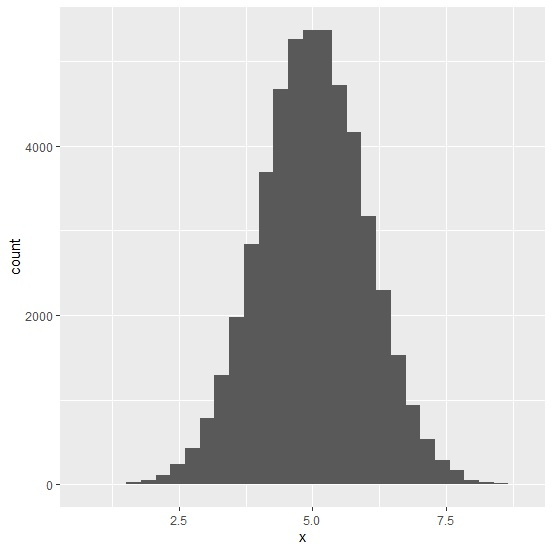# How to deal with warning message stat_bin() using bins = 30. Pick better value with binwidth. in R while creating a histogram?

The default value for bins is 30 but if we don’t pass that in geom_histogram then the warning message is shown by R in most of the cases. To avoid that, we can simply put bins=30 inside the geom_histogram() function. This will stop showing the warning message.

Consider the below data frame −

x<-rnorm(50000,5,1)
df<-data.frame(x)

## Example

library(ggplot2) ggplot(df,aes(x))+geom_histogram() stat_bin() using bins = 30. Pick better value with binwidth.

## OutputCreating the histogram by specifying the bins −

## Example

ggplot(df,aes(x))+geom_histogram(bins=30)

## OutputUpdated on: 18-Oct-2020

5K+ Views